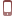# 初二数学，如何用中垂线和勾股定理求面积？考试用这种方法很管用手机版

点击右上角关注“良师益友谈育儿”分享学习经验，一起畅游快乐的学习生活。

中垂线是初二数学的重要知识点，利用中垂线的性质可以进行计算证明，本文就例题详细解析这类题型的辅助线作法和解题思路，希望能给初二学生的数学月考复习带来帮助。

例题

如图，在四边形ABCD中，对角线AC⊥BD，垂足为O，且∠OAB=45°，OC=2OA=8，∠OCB=1/2∠ODA，求四边形ABCD的面积。

解题过程：

根据题目中的条件：AC⊥BD，则∠AOD=∠AOB=∠BOC=∠COD=90°；

根据题目中的条件和结论：∠AOB=90°，∠OAB=45°，∠AOB+∠OAB+∠OBA=180°，则∠OBA=45°；

根据题目中的条件和结论：∠OAB=45°，∠OBA=45°，则∠OAB=∠OBA；

根据等边对等角的性质和结论：三角形中相等的角所对的边相等，∠OAB=∠OBA，则OA=OB；

根据题目中的条件和结论：2OA=8，OA=OB，则OA=OB=4；

取BC的中点E，过点E作EF⊥BC，交OC于点F，连接BF

根据题目中的条件：E是BC的中点，则BE=CE；

根据垂直平分线的性质和结论：线段垂直平分线上的点到线段两端距离相等，BE=CE，EF⊥BC，则BF=CF；

根据等边对等角的性质和结论：三角形中相等的边所对的角相等，BF=CF，则∠FBC=∠OCB；

根据外角的性质和结论：三角形的一个外角等于不相邻的两个内角和，则∠OFB=∠FBC+∠OCB；

根据结论：∠FBC=∠OCB，∠OFB=∠FBC+∠OCB，则∠OFB=2∠OCB；

根据题目中的条件：∠OCB=1/2∠ODA，则∠ODA=2∠OCB；

根据结论：∠OFB=2∠OCB，∠ODA=2∠OCB，则∠OFB=∠ODA；

根据三角形的判定和结论：两组对应角及一组角的对边分别相等的两个三角形全等，∠ODA=∠OFB，∠AOD=∠BOC，OA=OB，则△ODA≌△OFB；

根据全等三角形的性质和结论：全等三角形的对应边相等，△ODA≌△OFB，则OD=OF；

设OD=OF=x

根据题目中的条件：OC=8，OD=x，则CF=OC-OD=8-x；

根据结论：BF=CF，CF=8-x，则BF=8-x；

根据勾股定理和结论：∠BOC=90°，OB^2+OF^2=BF^2，OB=4，OF=x，BF=8-x，则4^2+x^2=(8-x)^2，可求得x=3；

根据结论：x=3，OD=OF=x，则OD=3；

根据三角形面积计算公式、题目中的条件和结论：OA=OB=4，OC=8，OD=3，则S△AOB=OA*OB/2=8，S△AOD=OA*OD/2=6，S△COD=OC*OD/2=12，S△BOC=OB*OC/2=16；

根据题目中的条件和结论：S△AOB=8，S△AOD=6，S△COD=12，S△BOC=16， 则S四边形ABCD=S△AOB+S△AOD+S△COD+S△BOC=42。

结语

解决本题的关键是合理添加中垂线构造出直角三角形和全等三角形，利用勾股定理列方程求解三角形的边长，再把四边形分割为四个直角三角形进行求解。这种方法在考试中经常用到，值得大家学习并掌握！